# How to Sum by Formula If Cells Are Not Blank in Criteria Range

Sometimes we may meet the cases that some blank cells exist in criteria range or sum range. In most situations we will ignore them, so we need to filter data by ‘not blank/not empty’. Today we will introduce you how can we sum only for not blank cells if some are blank in criteria range.

Through demonstrate a simple instance, we will introduce you the syntax, arguments of SUMIFS function, and let you know how the formula works step by step and finally reach your goal. After reading the article, you may have a simple understanding of SUMIFS function. We will also introduce you another method to resolve this issue by SUMIF function at the end.

## EXAMPLE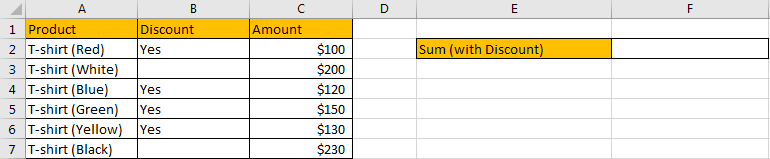Refer to above table, we can see that some different colors of T-shirt are listed in “Product” column. For these T-shirts, due to different colors, some of them are available at a discount. Amount for each color T-shirt is listed in “Amount” column accordingly.

In this instance, we want to calculate total amount for the products which have a discount on sale. So, we need to filter data in ‘Discount’ column based on the criteria that ‘discount field is not blank’. Then we can sum up filtered values in “Amount” column. To resolve this issue by formula, we can apply SUMIFS or SUMIF functions.

## FORMULA – SUMIFS FUNCTION

Step 1: Select B2:B7, then in Name Box define a new name for this range, for example ‘Discount’.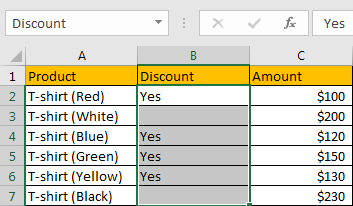Step 2: Select C2:C7, in Name Box define a new name for this range, for example ‘Amount’.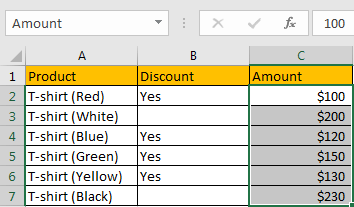Step 3: In E2, enter the formula =SUMIFS(Amount,Discount,”<>”).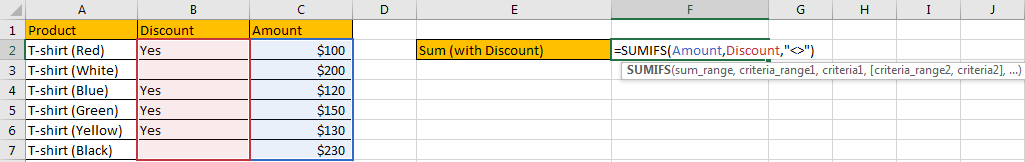NOTE: In step#1 and step#2 we defined range name “Discount” and “Amount”, when entering the formula, after typing “Amou…”, defined range “Amount” is auto loaded, you can directly select it from dropdown list. You can also select B2:B7, C2:C7 to fill formula arguments as well.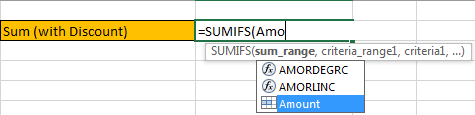Step 4: Press Enter after typing the formula. A number is returned.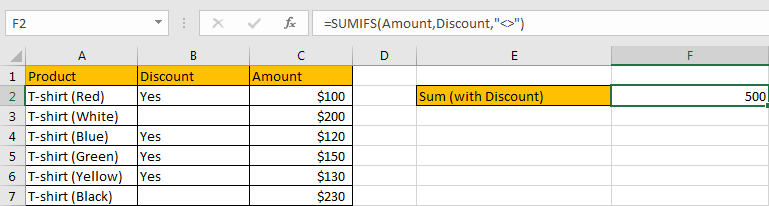We can see in column B, except B3 and B7, other cells are filled with ‘Yes’ and not empty, the corresponding amounts are 100 (in cell C2), 120 (in cell C4), 150 (in cell C5) and 130 (in cell C6), so the total amount is 100+120+150+130=500. The formula works correctly.

### SUMIFS FUNCTION INTRODUCTION

SUMIFS function can be seen as SUM+IFS, it can handle multiple ‘criteria range’ and ‘criteria’ combinations.

For SUMIFS function, the syntax is:

SUMIFS(sum_range, criteria_range1, criteria1, [criteria_range2, criteria2], …). Contents in [] are optional.

SUMIFS function supports wildcards like asterisk ‘*’ and question mark ‘?’, it also supports logical operators within its arguments. If wildcards or logical operators are required, they should be enclosed into double quotes (““) with text.

The usage of wildcards:

1. An asterisk (*) means one or more characters.
2. A question mark (?) means one character.
3. The position of asterisk or question mark means the character(s) position relative to the entered part. For example, “*A*” means characters or texts are both listed before and after “A”. “A*” means this text is started with A, but ends with others.

The usage of logical operators:

1. “>” – greater than
2. “<” – less than
3. “<>” – not equal to

SUMIFS ARGUMENTS EXPLANATION

SUMIFS – SUM RANGE

In our instance, C2:C7 is the ‘sum range’ obviously. We define this range with name ‘Amount’ in above step#2.

In the formula bar, select ‘Amount’, press F9, values in this range are listed in an array.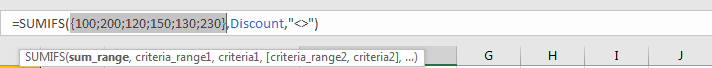SUMIFS – CRITERIA RANGE 1

We have only one criteria range in this case, it is B2:B7. This range records if product has a discount.

In the formula bar, select ‘Discount’, press F9, values in this range are listed in an array.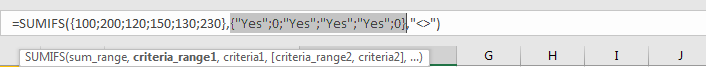SUMIFS – CRITERIA 1

We want to calculate total amount for the products which has a discount. As we mentioned above, SUMIFS function allows logical operators, so just enter “<>” as criteria. “<>” means not equal to, when it is used as criteria, it means “not empty”.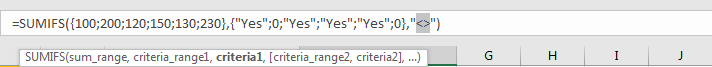HOW SUMIFS FORMULA WORKS

After explaining each argument in the formula, now we will show you how the formula works with these arguments.

Refer to above mentioned arguments, the formula is converted into below format in the formula bar.

=SUMIFS({100;200;120;150;130;230},{“Yes”;0;”Yes”;”Yes”;”Yes”;0},”<>”)

In the formula, there is one pair of criteria range and criteria:

Criteria Range: {“Yes”;0;”Yes”;”Yes”;”Yes”;0}

Criteria: “<>”

If cell is not empty, mark it in bold:

{“Yes“;0;”Yes“;”Yes“;”Yes“;0}

So, for bold texts, record a ‘True’ in the array; otherwise, record a ‘False’. Then we can get a new array:

{True;False;True;True;True;False}

Convert ‘True’ to ‘1’ and ‘False’ to ‘0’:

{1;0;1;1;1;0}

Now, we have below two arrays:

Sum Range: {100;200;120;150;130;230}

Criteria: {1;0;1;1;1;0} – if product has a discount, 1 is displayed.

We list the two arrays in two rows, multiply the two numbers in the same column, and save their products in another row, sum all products together, we can get 500 at last.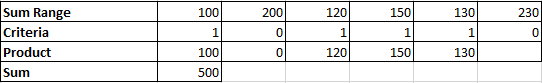1.In this case, as in ‘Discount’ column, only ‘Yes’ is recorded, you can also update the formula to “=SUMIFS(Amount,Discount,”Yes”)”.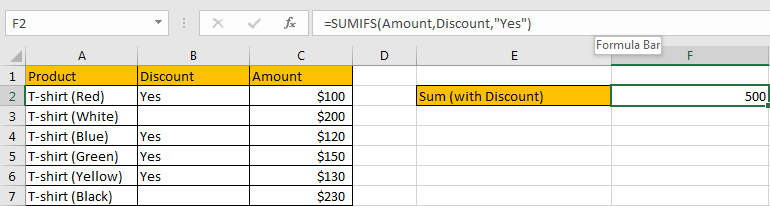2.The formula also works well if the discount is a real number.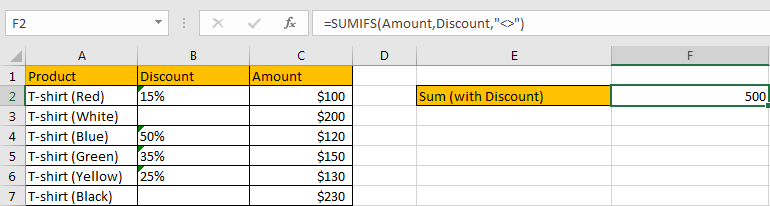3.In this case, we can also use SUMIF function if cells are not blank.

For SUMIF function, the syntax is:

=SUMIF (range, criteria, [sum_range]). Argument in [] is optional. If sum range is omitted, SUMIF will sum up all numbers in range argument. The order of the arguments is different from SUMIFS.

Enter the formula =SUMIF(Discount,”<>”,Amount).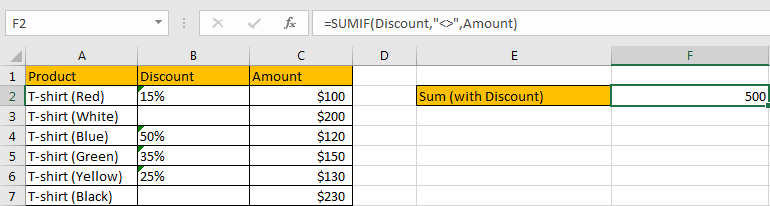After entering the formula, we can see that SUMIF function also works correctly. Actually, in most situations, SUMIF function can be used instead of SUMIFS function if there is only one pair of criteria range and criteria. If multiple criteria are supplied, you can choose SUMIFS to handle them.

SUMMARY

1. SUMIFS function can handle multiple groups of criteria ranges and criteria. Sum range is the first argument among all arguments.

2. It supports user defined range name.

3. It supports wildcard.

4. It supports logical operators. ”<>” can represent “not equal to/not empty” in a formula.

### Related Functions

• Excel SUMIFS Function
The Excel SUMIFS function sum the numbers in the range of cells that meet a single or multiple criteria that you specify. The syntax of the SUMIFS function is as below:=SUMIFS (sum_range, criteria_range1, criteria1, [criteria_range2, criteria2], …)…
• Excel SUMIF Function
The Excel SUMIF function sum the numbers in the range of cells that meet a single criteria that you specify. The syntax of the SUMIF function is as below:=SUMIF (range, criteria, [sum_range])…